# How to Filter Cells Starts with Number or Letter in Excel

This post will guide you how to filter values starts with number or letter in one single cell in Excel. How do I filter in a list those that begin with number or letter using a formula in Excel.

## Filter Cells Starts with Number or Letter

Assuming that you have a list of data in range B1:B4 which contain text string values. Ad you want to filter those cells values which are starting from a number or letter. The below steps will show you how to filter the data which starts with numbers or letters only.

Step1: select one blank cells in the adjacent Cell B1. And enter the following formula based on the ISNUMBER function and the LETF function.

`=ISNUMBER(LEFT(B1)*1)`

Note: Cell B1 is the first cell in your data which you want to filter out values that starts with number or letter.

Step2: press Enter key to apply this formula.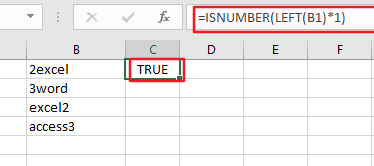Step3: then drag the AutoFill Handle in Cell C1 down to other cells to apply the same formula. You would see that if the data starts with a number, then returns TRUE. Otherwise, returns FALSE.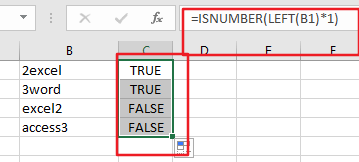Step4: select the cell C1 in the helper column, go to Data tab in the Excel Ribbon, and click Filter button under Sort & Filter group. And one Filter icon will be added into the Cell C1.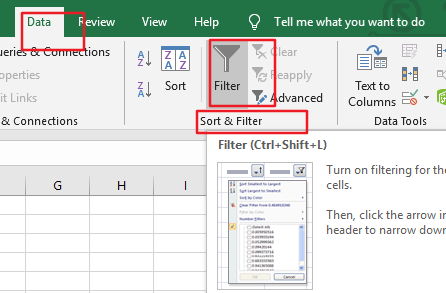Step5: click filter icon in the Cell C1, and check True to filter out all data that starts with number. Click Ok button.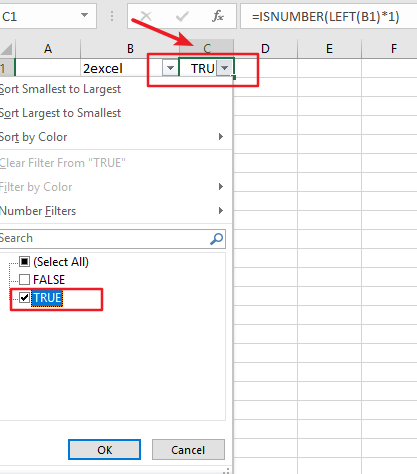Step6: let’s see the last result: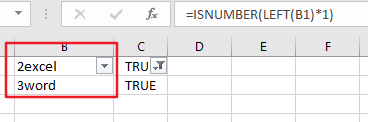### Related Functions

• Excel ISNUMBER function
The Excel ISNUMBER function returns TRUE if the value in a cell is a numeric value, otherwise it will return FALSE.The syntax of the ISNUMBER function is as below:= ISNUMBER (value)…
• Excel LEFT function
The Excel LEFT function returns a substring (a specified number of the characters) from a text string, starting from the leftmost character.The LEFT function is a build-in function in Microsoft Excel and it is categorized as a Text Function.The syntax of the LEFT function is as below:= LEFT(text,[num_chars])…

Related Posts

Abbreviate Names Or Words in Excel

As an MS Excel user, you might have come across a task where you need to abbreviate different names or words, and there are also possibilities that you might have done this task manually by assuming that there isn't any ...

Filter with Multiple Criteria

If you are an valid MS Excel user, you have probably come across a situation where you wanted to filter the data in a separate table with complex criteria. You could do this task manually, which is also acceptable while ...

Extract substring In Excel

This post will guide you how to use Excel's MID function is a quick and easy way to extract pieces from your text. Use the Excel formula to extract a substring with MID. Note: If you want to extract just ...

How to Split Cells by the First Space in Texts in Excel

Sometimes we may use space to separate texts to different groups in one cell, if we want to split this cell to multiple columns refer to space, we can implement this via ‘Text to Columns’ feature. But is there any ...

How to Convert Uppercase to Lowercase Except the First Letter in Excel

In excel, words can be entered in uppercase, lowercase or mixed. If we want to convert all uppercase to lowercase (except the first letter in some situations), we can use formula with some letter convert related functions to convert them ...

How to Remove Middle Name from Full Name in Excel

When we get a list of full names, we can remove the middle name for short in some cases. As the middle names are different among the full name list so we cannot replace them by space directly in excel. ...

How to Convert Text to Time in Excel

This post will guide you how to convert time string to time in Excel. Assuming that you have a list of text string in your worksheet, and you wish to convert these time string to standard time format, how to ...

How to Extract Initials From a Name in Excel

This post will guide you how to get initials from a given name using a formula in Excel. How do I extract initials from names in Excel 2013/2016. Extract Initials from a Name Using a Formula Extract Initials from a ...

How to Remove Last or Trailing Commas in Excel

This post will guide you how to remove trailing commas from cell in Excel. How do I remove comma character at the end of a cell with a formula in Excel 2013/2016. Remove Trailing Commas if you want to remove ...

How to Convert mmddyy to Date in Excel

This post will guide you how to convert non-standard date formats or text to a standard date in Excel. If you have a date with mmddyy format, and you want to change it as the normal date format as mm/dd/yyyy ...

Sidebar